# Auto-regressive process

(diff) ← Older revision | Latest revision (diff) | Newer revision → (diff)

A stochastic process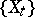whose values satisfy an auto-regression equation with certain constants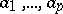:(*)

whereis some positive number and where the variables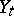are usually assumed to be uncorrelated and identically distributed around their average value 0 with a variance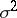. If all the zeros of the function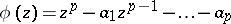of a complex variablelie inside the unit circle, then equation (*) has the solutionwhere theare connected with theby the relation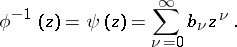For example, let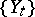be a white noise process with spectral density; in such a case the only kind of auto-regressive process satisfying equation (*) will be a processwith spectral density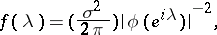which is stationary in the wide sense if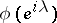has no real zeros. The autocovariances (cf. Autocovariance)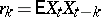of the process satisfy the recurrence relation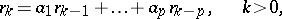and, in terms of the, have the formThe parametersof the auto-regression are connected with the auto-correlation coefficients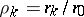of the process by the matrix relationwhere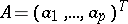,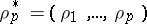and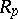is the matrix of auto-correlation coefficients (the Yule–Walker equation).

How to Cite This Entry:
Auto-regressive process. A.V. Prokhorov (originator), Encyclopedia of Mathematics. URL: http://encyclopediaofmath.org/index.php?title=Auto-regressive_process&oldid=15186
This text originally appeared in Encyclopedia of Mathematics - ISBN 1402006098